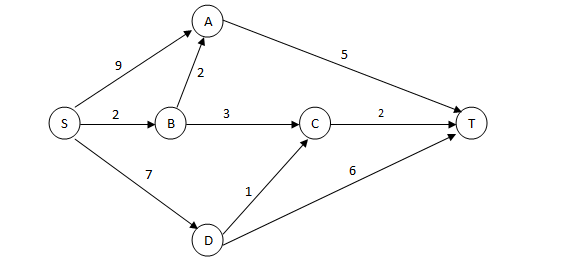### CAT 2006 Question Paper Question 45

Instructions

A significant amount of traffic flows from point S to point T in the one-way street network shown below. Points A, B, C, and D are junctions in the network, and the arrows mark the direction of traffic flow. The fuel cost in rupees for travelling along a street is indicated by the number adjacent to the arrow representing the street. –Motorists traveling from point S to point T would obviously take the route for which the total cost of traveling is the minimum. If two or more routes have the same least travel cost, then motorists are indifferent between them. Hence, the traffic gets evenly distributed among all the least cost routes.

The government can control the flow of traffic only by levying appropriate toll at each junction. For example, if a motorist takes the route S-A-T (using junction A alone), then the total cost of travel would be Rs 14 (i.e., Rs 9 + Rs 5) plus the toll charged at junction A.

Question 45

# The government wants to devise a toll policy such that the total cost to the commuters per trip is minimized. The policy should also ensure that not more than 70 per cent of the total traffic passes through junction B. The cost incurred by the commuter travelling from point S to point T under this policy will be:

Solution

The costs of the routes are as given below:
S - B - C - T = 7
S - B - A - T = 9
S - D - C - T = 10
S - D - T = 13
S - A -T = 14
Hence now 100% of the traffic flows through S - B - C - T
Now if we make the cost of traveling through S - B - C - T same as some other route not going through B, then the traffic will be equally distributed between these two routes. The lowest such route is S-D-C-T. The difference in cost = 3. Hence if we levy a toll of Rs.3 at B, the costs of SBCT and SBAT become 10,12 respectively and other routes are not affected. So 50% traffic flows through SBCT and 50% flows through SDCT . Hence cost in this policy = 10.